# Posts tagged "Excel functions", page 6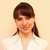## INDEX function in Excel - 6 most efficient uses

In this tutorial, you will find a number of Excel INDEX formula examples that demonstrate the most efficient uses of INDEX in Excel. Continue reading## Using OFFSET function in Excel - formula examples

The tutorial explains the arcane twists of the OFFSET function and covers the most efficient ways to use OFFSET in Excel. Continue reading## How to use INDIRECT function in Excel - formula examples

This Excel INDIRECT tutorial explains the function's syntax, basic uses and provides a number of formula examples that demonstrate how to use INDIRECT in Excel. Continue reading## How to calculate compound interest in Excel: daily, monthly, yearly compounding

The tutorial explains the compound interest formula for Excel and provides examples of how to calculate the future value of the investment at annual, monthly or daily compounding interest rate. You will also find the detailed steps to create your own Excel compound interest calculator. Continue reading## Using logical functions in Excel: AND, OR, XOR and NOT

Last week we tapped into the insight of Excel logical operators that are used to compare data in different cells. Today, you will see how to extend the use of logical operators and construct more elaborate logical tests to perform more complex calculations and more powerful data analysis. Excel logical functions such as AND, OR, XOR and NOT will help you in doing this. Continue reading## Excel logical operators: equal to, not equal to, greater than, less than

Many tasks you perform in Excel involve comparing data in different cells. For this, Microsoft Excel provides six logical operators, which are also called comparison operators. This tutorial aims to help you understand the insight of Excel logical operators and write the most efficient formulas for your data analysis. Continue reading## Excel IF statement with multiple conditions

For powerful data analysis, you may often need to build an Excel IF statement with multiple conditions or use IF together with other functions. This tutorial will show you the most effective ways to do this. Continue reading## How to use IF function in Excel: examples for text, numbers, dates, blanks

IF is one of the most popular and useful functions in Excel. Generally, you use an IF statement to test a condition and to return one value if the condition is met, and another value if the condition is not met. Continue reading## How to use Excel SUMIFS and SUMIF with multiple criteria

This tutorial explains the difference between the SUMIF and SUMIFS functions in terms of their syntax and usage, and provides a number of formula examples to sum values with multiple AND / OR criteria in Excel. Continue reading## How to use SUMIF function in Excel with formula examples

If you are faced with a task that requires conditional sum in Excel, the SUMIF function is what you need. It is a really great function that can help you make sense of an incomprehensible set of diverse data. Instead of adding up all numbers in a range, it lets you sum only those values that meet your criteria. Continue reading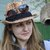## 5 ways to sum a column in Excel

This tutorial shows how to sum a column in Excel 2010-2013. Try out 5 different ways to total columns: find the sum of the selected cells on the Status bar, use AutoSum in Excel to sum all or only filtered cells, employ the SUM function or convert your range to Table for easy calculations. Continue reading## How to convert numbers to text using Excel TEXT function and other options

This tutorial shows how to convert number to text in Excel 2016 - 2010. See how to accomplish the task with the Excel TEXT function and use number to string to specify the formatting. Learn how to change number format to text with the Format Cells… and Text to Columns options. Continue reading## Excel VLOOKUP not working - solving #N/A, #NAME, #VALUE errors

The tutorial explains how you can quickly cope with VLOOKUP not working problems in Excel, troubleshoot and fix common errors and overcome VLOOKUP's limitations. Continue reading## 5 ways to do case-sensitive VLOOKUP in Excel

The tutorial explains how to make Excel VLOOKUP case-sensitive, demonstrates a few other formulas that can look up case in Excel and points out strengths and limitations of each function. Continue reading## INDEX & MATCH in Excel - better alternative to VLOOKUP

This tutorial demonstrates the key strengths of Excel's INDEX / MATCH function that make it superior to VLOOKUP. You will find a number of formula examples that will help you easily cope with many complex tasks when VLOOKUP fails. Continue reading## How to use VLOOKUP with SUM or SUMIF functions in Excel

In this tutorial, you will find a handful of advanced formula examples that demonstrate how to use Excel's VLOOKUP and SUM or SUMIF functions to look up and sum values based on one or several criteria. Continue reading## Advanced VLOOKUP in Excel: multiple, double, nested

In this VLOOKUP tutorial, you will find a number of advanced formula examples that demonstrate how to look up with multiple criteria, use two VLOOKUP functions in one formula, dynamically pull data from different sheets, and more. Continue reading## Excel VLOOKUP tutorial for beginners with formula examples

This VLOOKUP tutorial explains the syntax and provides a number of examples that illustrate the most common usages of the VLOOKUP function in Excel. Continue reading## How to use Excel COUNTIFS and COUNTIF with multiple criteria

The tutorial explains how to use COUNTIFS and COUNTIF formulas with multiple criteria in Excel. You will find a number of examples for different data types – numbers, dates, text, wildcard characters, non-blank cells and more. Continue reading## COUNTIF function in Excel - count if not blank, greater than, duplicate or unique

The article explains Excel COUNTIF function, provides a number of examples and warns about possible quirks when using COUNTIF with multiple criteria and specific types of cells. Continue reading## 3 ways to count empty cells in Excel

If your task is to get Excel count blank cells in your worksheet, read this article to find 3 ways to accomplish it. Learn how to search and select empty cells with the Go to Special option, use Find and Replace to count blanks or enter a formula in Excel 2010-2013. Continue reading## How to count non-empty cells in Excel

This articles looks at the ways to count non-blank cells in Excel 2010-2013. Below you'll find 3 methods for counting non-blanks: see the number on the Excel Status bar, employ the Find and Replace dialog or use a special formula. Continue reading## Excel conditional formatting formulas based on another cell

This tutorial explains how to use Excel formulas to format cells and entire rows based on the values you specify or based on another cell's value, and provides a handful of formula examples. Continue reading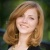## How to add a hyperlink to another worksheet

In this article I'll show you 3 ways how you can add hyperlinks into your Excel workbook to easily navigate between numerous worksheets. You'll also learn how to change a link destination and modify its format. If you don't need a hyperlink any more, you'll see how to quickly remove it. Continue reading## 4 ways for changing case in Excel

In this article I'd like to tell you about different ways to change Excel uppercase to lowercase or proper case. You'll learn how to perform these tasks with the help of Excel lower/upper functions, VBA macros, Microsoft Word, and an easy-to-use add-in by Ablebits. Continue reading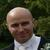## 6 Reasons Why Your VLOOKUP is Not Working

The VLOOKUP function is the most popular lookup and reference function in Excel. It is also one of the trickiest and the dreaded #N/A error message can be a common sight. This article will look at the 6 most common reasons why your VLOOKUP is not working. Continue reading## How to convert rows to columns in Excel (transpose or rotate data)

In this article, you will find several ways to convert rows to columns and columns to rows in Excel. These solutions work in all versions of Excel 2016, 2013, 2010, 2007 and lower, cover many possible scenarios, explain most typical mistakes and provide good examples of using Excel's Transpose function. Continue reading## How to sum and count cells by color in Excel

Even though Microsoft Excel has a variety of functions for different purposes, none can count or sum by color of a cell. Aside from third-party tools, there is only one possible solution - create your own functions. Continue reading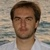## How to quickly extract domain names from URLs in Excel

A few tips and pieces of advice will help you get domain names from a list of URLs using Excel formulas. Two variations of the formula let you extract the domain names with and without www. regardless of the URL protocol (http, https, ftp etc. are supported). The solution works in all modern versions of Excel, from 2007 through 2016. Continue reading## How to compare two columns in Excel and delete duplicates (highlight, color, move)

It will take you about 10 minutes to read this article and in the next 5 minutes (or even faster if you choose the 2nd solution described in the article :) you will easily compare two Excel columns for duplicates and remove or highlight the found dupes. Okay, the countdown is started! Continue reading